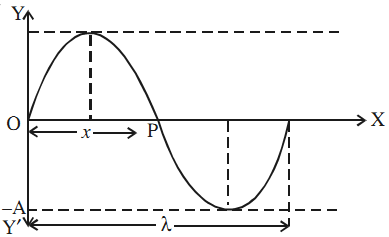# Equation of Simple Harmonic Wave in One Dimension

Consider a simple harmonic wave propagating along OX. Assume that the wave is transverse and the vibrations of the particle are along YOY′.The displacement at t = 0 is given by the equation

y = a sin ωt

The phase of vibrations at that time at the point P lags behind by a phase, say φ. Then

y = a sin (ωt – φ)

Let OP = x. Since phase change per unit distance is k,

φ = kx.

Hence,

y(x, t) = a sin (ωt – kx)

As ω = 2π/t and k = 2π/λ,

y(x, t) = a sin 2π (t/T – x/λ)

In terms of wave velocity (v = λ/T), this equation can be expressed as

y = a sin 2π/λ (vt – x)

If the initial phase angle at O is φ0, the equation of the wave would be

y(x, t) = a sin [(ωt – kx) + φ0]

Phase difference between two points on a wave

Consider two simple harmonic waves travelling along OX and represented by the equations

y = a sin (ωt – kx)

y = a sin [ωt – k(x + ∆x)]

The phase difference between them is

∆φ = k∆x = 2π/λ . ∆x = –2π/λ (x2 – x1)

where ∆x is called the path difference between these two points. The negative sign indicates that a point positioned later will acquire the same phase at a later time.

Phase difference at the same position over a time interval ∆t

Consider two waves at the same position at a time interval ∆t.

∆φ = 2πf(∆t)